1. Four paversFour pavers would pave the square in 18 days. How many pavers do you need to add to done work in 12 days?
2. Six percents6% of the base is 21. How much is 28% of this base?
3. Percentage increaseIncrease number 400 by 3.5%
4. Shopping center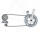The shopping center buys from the manufacturer bikes at a purchase price of 180 €. It sells them for a sale price of 250 €. However, in the advertising for the sale of these goods, the shopping center spent 20% of the selling price of all bicycles in stoc
5. Sand castle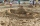Tim and Tom built a sand castle and embellished it with a flag. Half the pole with the flag plunged into the castle. The highest point of the pole was 80 cm above the ground, its lowest point 20 cm above the ground. How high was the sand castle?
6. The sides 2The sides of a trapezoid are in the ratio 2:5:8:5. The trapezoid’s area is 245. Find the height and the perimeter of the trapezoid.
7. Find the 9Find the missing angle in the triangle and then name triangle. Angles are: 95, 2x+15, x+3The school received money from the sponsor. She used one-third to buy computers, half of the rest to adjust the gym. It still has 4000 euros left. How many euros did the sponsor donate to the school?
9. The workThe work was to be done by 150 workers. At the beginning of their work, their number reduced by 40, which increased the time of work by 5 and 1/3 of the schedule. How long did work take?
10. Trapezium diagonalsIt is given trapezium ABCD with bases | AB | = 12 cm, |CD| = 8 cm. Point S is the intersection of the diagonals for which |AS| is 6 cm long. Calculate the length of the full diagonal AC.
11. Four workers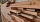Four workers will lay the floor of the gym in six hours. After an hour of working together, one of the workers left to the doctor. How long before the remaining three workers finish their work?
12. MG=7x-15,MG=7x-15, FG=33, x=? Point M is the midpoint of FG. Find unknown x.
13. Midpoint 6FM=8a+1, FG=42, a=? Point M is the midpoint of FG. Find unknown a.
14. Find midpoint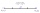FM=5y+13, MG=5-3y, FG=? M is the midpoint of FG. Use the given information to find the missing measure or value.
15. Midpoint 5FM=3x-4, MG=5x-26, FG=? Point M is the midpoint of FG. Use the given information to find the missing measure or value.
16. 3rd dimensionThe block has a surface of 42 dm2 and its dimensions are 3 dm and 2 dm. What is the third dimension?
17. Horizontal distance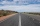The road has a gradient of 8%. How many meters will the road rise on a horizontal distance of 400m?
18. Eight hoursBrigadier Franta ties a sheaf of hay in 20 minutes. Lujza in the same time only a quarter. How many sheaves will they tie together in 8 hours?
19. Three-digit integersHow many three-digit natural numbers exist that do not contain zero and are divisible by five?
20. Coins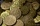The boy collected coins with value 5 CZK and 2 CZK when he had 50 pieces saved 190 CZK. How many he has each type of coin?

Do you have an interesting mathematical word problem that you can't solve it? Submit math problem, and we can try to solve it.

We will send a solution to your e-mail address. Solved examples are also published here. Please enter the e-mail correctly and check whether you don't have a full mailbox.

Please do not submit problems from current active competitions such as Mathematical Olympiad, correspondence seminars etc...